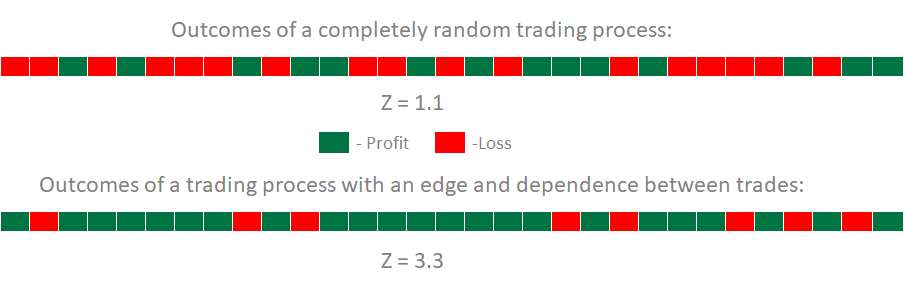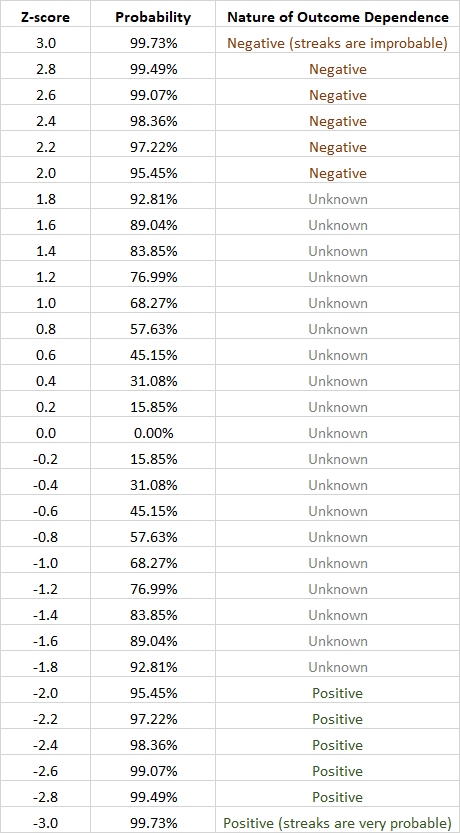# Z-Score Optimization in Forex Trading

This guide sheds light on a very important but often overlooked concept in EA optimization — Z-score. But before we describe the actual use case of Z-score optimization, it would be wise to explain a bit more about this interesting trading system performance indicator.

## What is Z-score?

A Z-score (or standard score) measures the distance between the mean of some set of the statistical results and the given observation.

In Forex systems, traders are interested in Z-score not of a trade's return (profit/loss size) but rather in a Z-score of the outcome — was it a profitable one or a losing one? A Z-score, calculated using winning and losing streaks, measures the dependence between the outcome of the previous position and the outcome of the next position.

If we consider profitable positions as positive results and losing positions as negative statistical results, counting the total number of all wins, losses, overall trades, as well as the number of win and loss streaks, we can calculate a Z-score for a given trading strategy or expert advisor.

Usually, a Z-score fluctuates between -3 to +3, but sometimes, can go above and below these "limits". A Z-score value of 0 means that we are dealing with completely random results.

Each Z-score value has also a probability of dependence (P) associated with it, which informs us of how probable dependence between the trades is. P values below -2 and above +2 have high (>95%) probability of dependence between trades:

• A positive Z-score means that profitable position is likely to be followed by a losing one, while a losing one should probably be followed by a winning one. Basically, it means that the probability of long winning and losing streaks is low.
• A negative Z-score means that profitable positions are likely to be followed by more profitable positions, and losing positions are to be followed by more losing positions. Basically, it means that winning or losing streaks are probable.

The concept of Z-score is illustrated in this image of two samples. The first sample is taken from a completely random trading system (50% chance of winning and 50% chance of losing), and the second sample is taken from a trading system with significant edge and dependence on previous trades:As you can see, their Z-scores are totally different, with a large positive Z-score of the second system signaling high likelihood of switching between profitable and losing trades.

## How to calculate Z-score of Forex system

To calculate a Z-score of your system, you need a rather large sample of trade outcomes recorded in chronological order. Using these outcomes, you can calculate Z-score with this formula:where:

• Z is the Z-score you are calculating;
• N is the total number of trades in the sample;
• R is the total number of losing and winning streaks;
• P equals 2 × W × L;
• W is the total number of winning trades in the sample;
• L is the total number of losing trades in the sample.

You can see your Z-score by loading your MT4 or MT5 trading report into our Forex report analyzer.

### Example of Z-score calculation

Let's look at the following example calculation process of a system's Z-score.

Let's assume a sample of 100 trading outcomes with 40 streaks (winning and losing), 50 winning trades, and 50 losing trades; then:

• N = 100;
• R = 40;
• P = 2 × 50 × 50 = 5000.The negative Z-score value of -2.11 means that the system's trading outcomes are likely non-random and that losing positions will likely be followed by more losing positions while profitable trades will likely be followed by more profitable trades (trades are likely to come in streaks).

### Z-score probability

Each Z-score value has a certain probability associated with it. This probability tells us how likely is it that the system that we analyze produces outcomes that are dependent on the previous outcome.

You can look at this table to quickly find the probability of such a connection between your trading results if you already know your Z-score:If we consider the Z-score value of -2.11 from the example above, we can see that the probability of positive dependence between the system's outcomes is between 95.45% and 97.22%, which is quite significant.

## How to use Z-score in Forex?

If you know the Z-score of your expert advisor or trading system and its value is above +2 or below -2, you can "skip" a trade when a losing position is expected. Nevertheless, the profit on this "skipped" position should be tracked (virtually), to know when to start trading again.

It should also be noted that Z-score calculation makes sense only for sufficiently large samples. Math literature suggests a sample size of no less than 51 to get a reliable Z-score value.

## Z-score optimization

For example, you backtested your expert advisor and found out that after more than a hundred trades, the resulting Z-score is +3.02, which means that profitable and losing positions are likely to appear in an alternating order (long streaks of losses or wins are not probable). The probability for Z-score value of +3.02 is greater than 99.73%.

It would make sense to modify the code of such an expert advisor to stop sending live orders when a profitable position is closed (the next one is very likely to be a loss). The EA would then enter a sort of a virtual trading mode, where position is opened and tracked only virtually (using MQL4 variables). When such position is closed (virtually of course), its profit/loss is considered — if it is a loss, live trading becomes enabled once again; if it is a win, operation in virtual mode should be continued. This is how it should be done for positive Z-scores.

Of course, it can be easily modified to work with negative Z-score.

You can download an example MT5 EA with positive Z-score optimization implemented:

## Further improvement

This scheme of Z-score optimization implies that you measure your Z-score on a backtest and then consider that it won't change much during the live run in future. An alternative plan would be to implement a constant measurement of Z-score inside your EA's code and to adjust the optimization on-the-fly. The problem is that it is quite difficult to implement this in a live expert advisor.

When the shares of the winning and losing positions are very unequal, Z-score optimization may become a bit tricky. In this case, it is probably better to make it asymmetrical (favoring less rare outcomes) or to skip it completely, even if Z-score is above/below +2/-2.

One notable case where Z-score optimization would be very inefficient and even ruinous is when your expert advisor opens more than one trade at once and these trades have nearly identical chance of ending up in loss or in profit. If your EA trades like that, you need to count such a group of nearly simultaneous trades as one outcome when computing a Z-score.

If you have any questions regarding the use of a Z-score metric in expert advisor optimization or if you want to share some idea for using Z-score values in Forex trading, you can start a discussion on our forum.

If you want to get news of the most recent updates to our guides or anything else related to Forex trading, you can subscribe to our monthly newsletter.